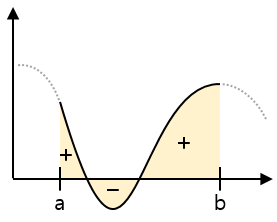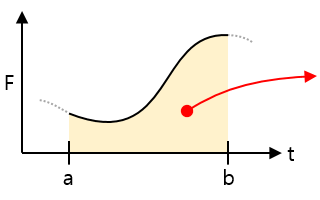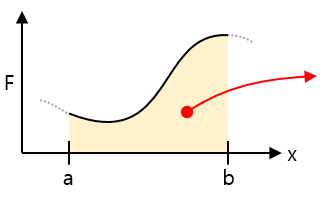# Integral in physics

## Definite integral

In the 2D coordinate plane, a definite integral is a way to find the area of a graph.

In a rectangle, the only simple way to get the area is the multiplication of width and height.
If the shape is not constant, you can not get the area by simple multiplication. When a section of the graph is defined, we use a definite integral to find that section’s area.The mathematical definition of the integral is :

Try to divide the sections so that the width of the sections is infinitely small. When this sum is always close to a constant value, we define that value as the definite integral of a to b.
$(Area) =\, \int_{a}^{b} f(x) \; dx$

## How to definite integral

To integrate, you first need to know the derivative.
$\int_{a}^{b} f(x) \; dx \, = \, F(b) – F(a)$
 Speed-time graphArea = Distance traveled for the duration of the interval Acceleration-time graphArea = The rate of change of speed over the duration of the interval Force-time graphArea = The amount of received impact (momentum) during the time of the interval Force-distance graphArea = The amount of work done to move the distance of the section, the energy stored or released in the spring